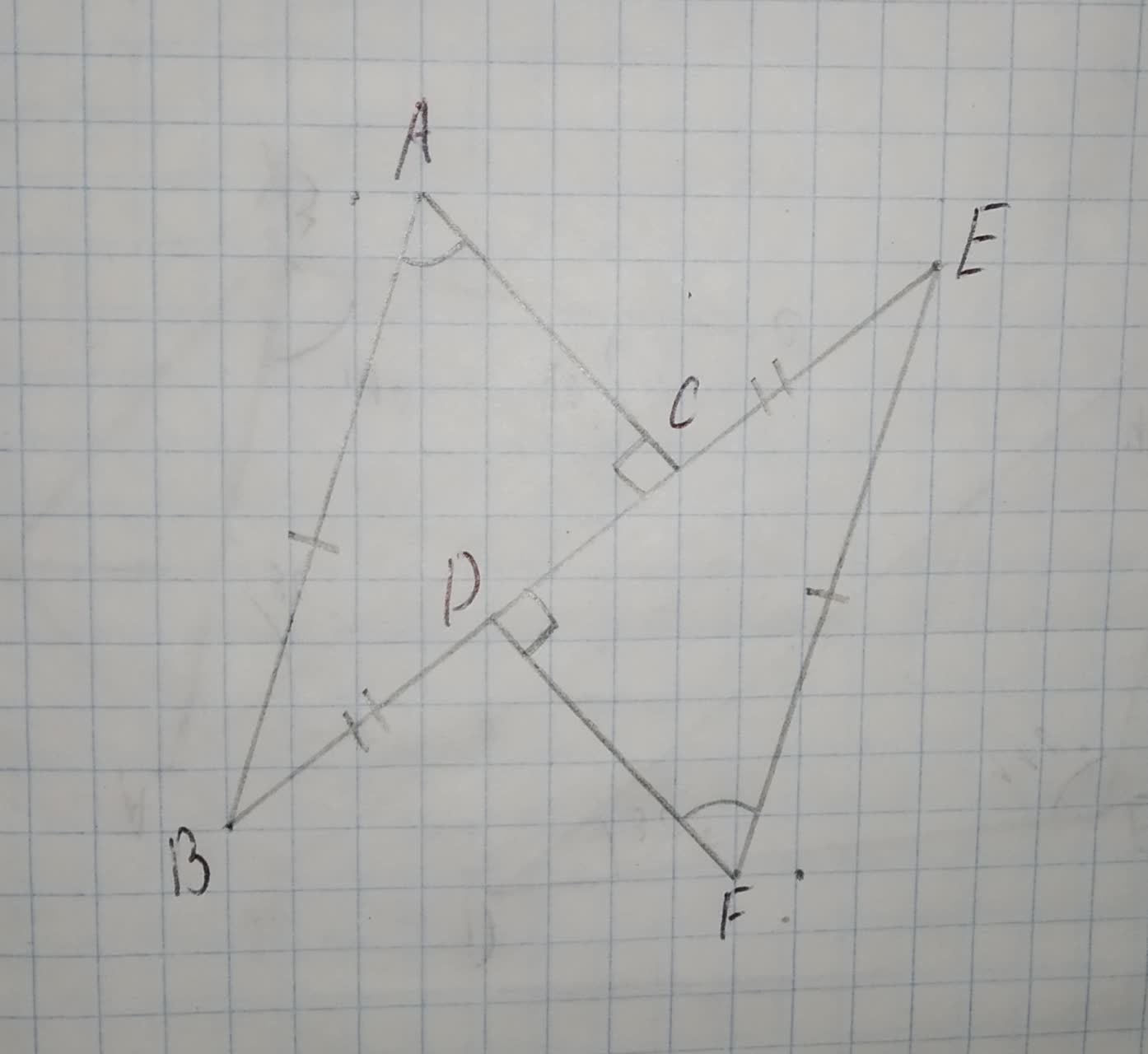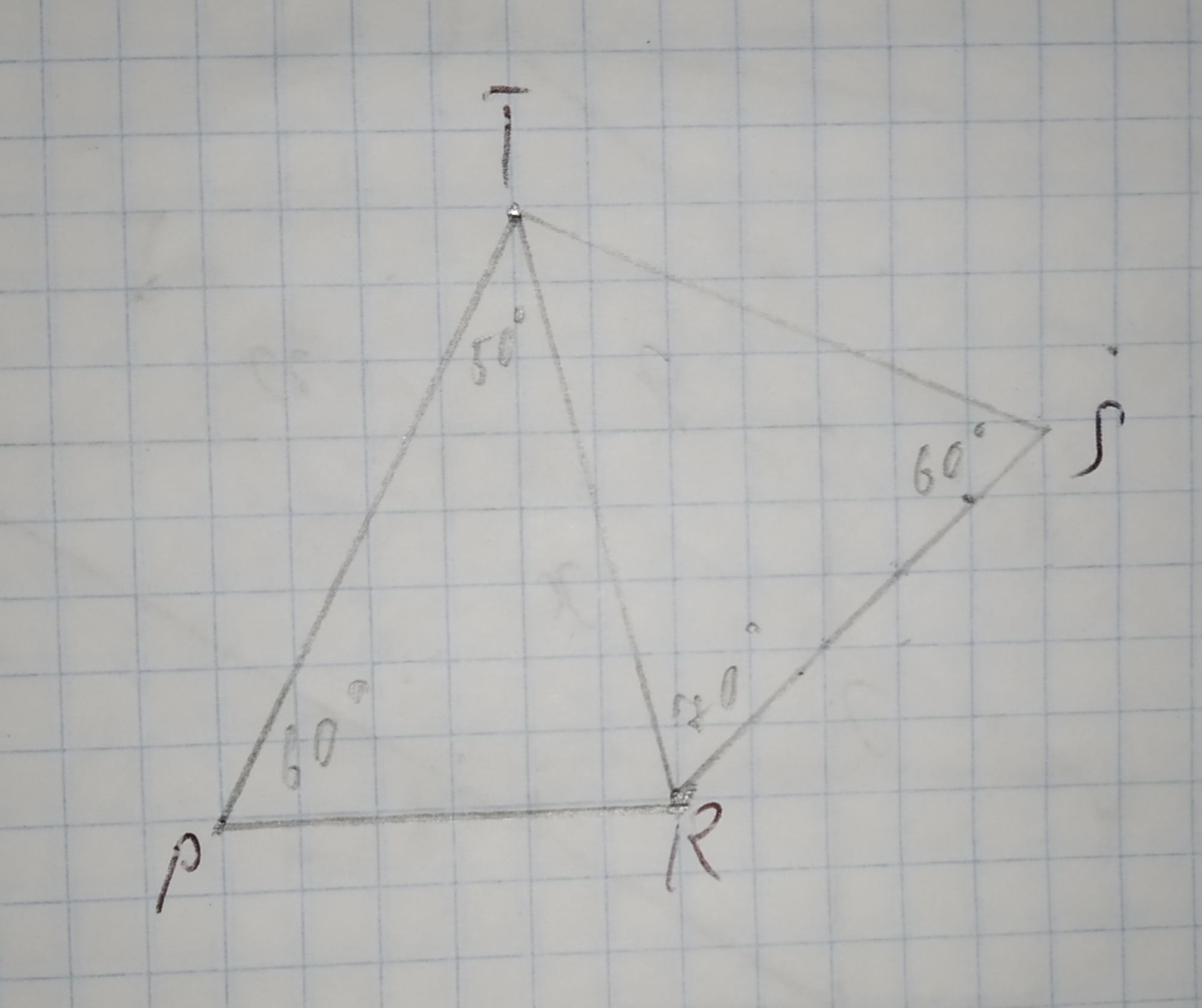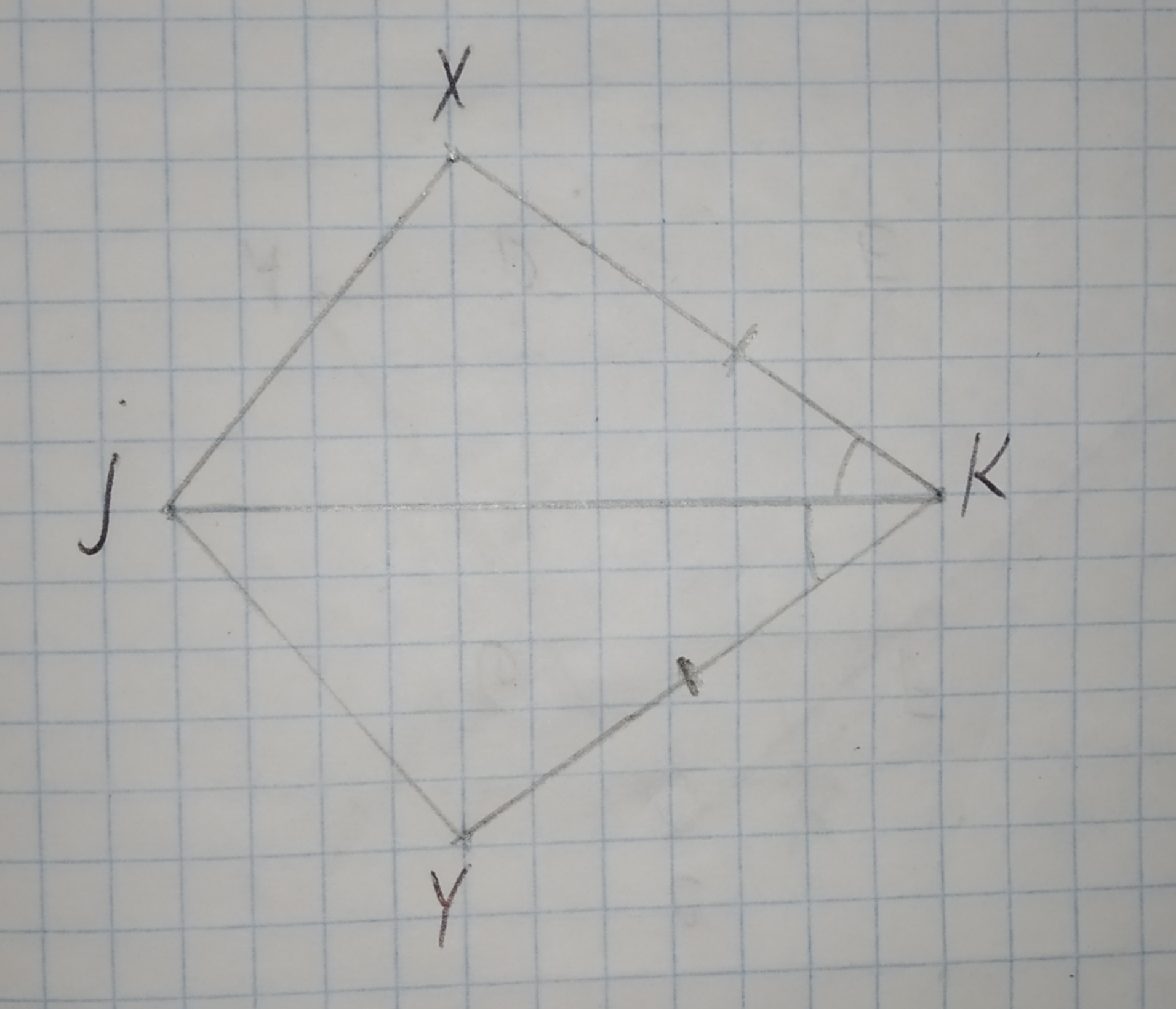# With of the following triangle congruence shortcuts could be used to prove PRQ = TRS 12210203401.jpg Given Data, /_Q ~= /_S bar(QR) ~= bar(SR) a)Side-Side-Side Postulate (SSS) b)Side-Angle-Side Postulate (SAS) c)Angle-Side-Angle Postulate (ASA) d)Angle-Angle-Side Theorem (AAS)Question
CongruenceWith of the following triangle congruence shortcuts could be used to prove PRQ = TRS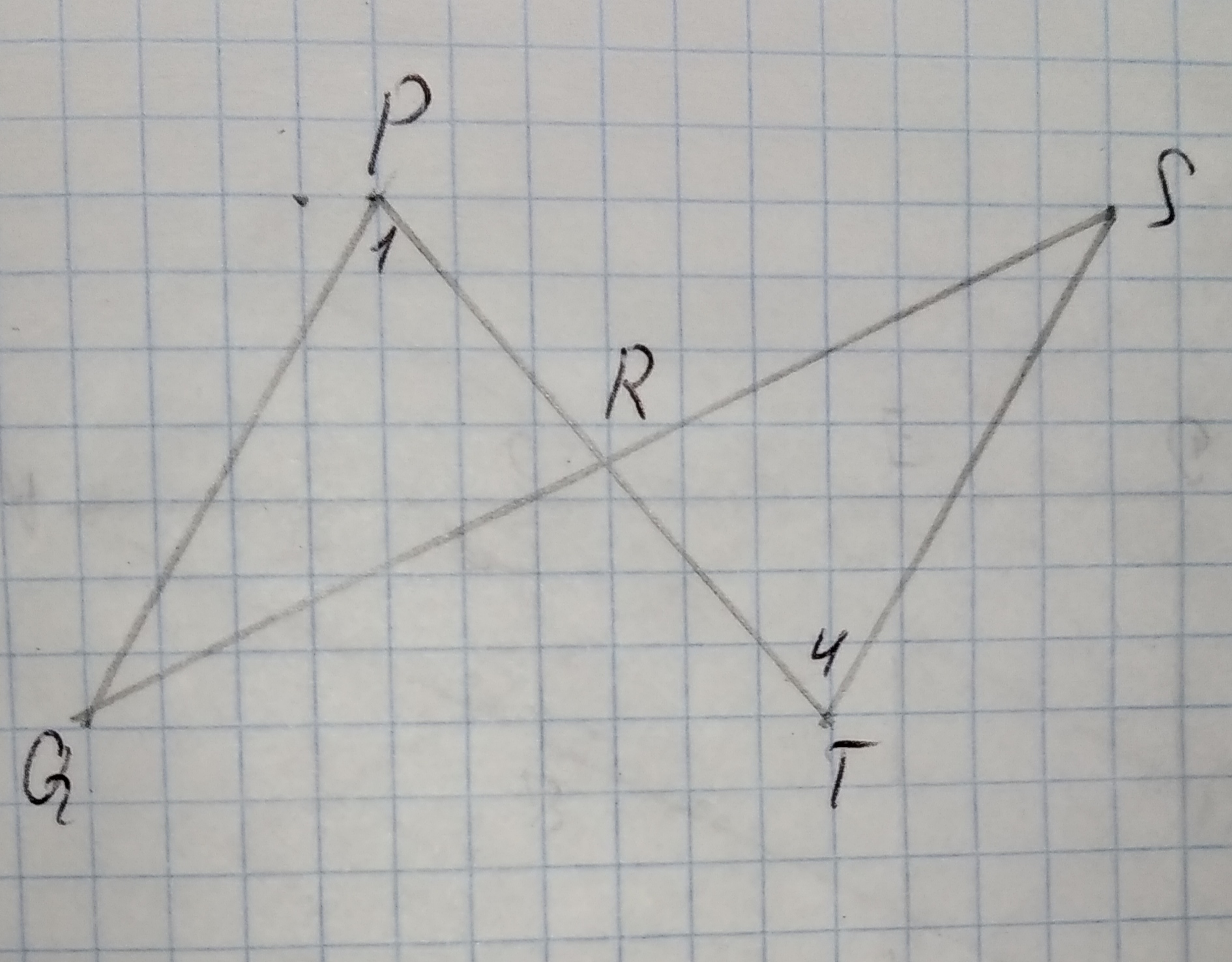Given Data,
$$\displaystyle\angle{Q}\stackrel{\sim}{=}\angle{S}$$
$$\displaystyle\overline{{{Q}{R}}}\stackrel{\sim}{=}\overline{{{S}{R}}}$$
a)Side-Side-Side Postulate (SSS)
b)Side-Angle-Side Postulate (SAS)
c)Angle-Side-Angle Postulate (ASA)
d)Angle-Angle-Side Theorem (AAS)2020-12-07
Step 1
It is given that,
$$\displaystyle\angle{Q}\stackrel{\sim}{=}\angle{S}$$
$$\displaystyle\overline{{{Q}{R}}}\stackrel{\sim}{=}\overline{{{S}{R}}}$$
$$\displaystyle{I}{n}\triangle{P}{Q}{R}{\quad\text{and}\quad}\triangle{R}{S}{T}$$,
$$\displaystyle\angle{Q}\stackrel{\sim}{=}\angle{S}$$ (equal angles given)
$$\displaystyle\overline{{{Q}{R}}}\stackrel{\sim}{=}\overline{{{S}{R}}}$$ ( equal sides given)
$$\displaystyle\angle{P}{R}{Q}\stackrel{\sim}{=}\angle{S}{R}{T}$$ ( vertically opposite angles)
Here, two angles and one side of both triangle is equal then,
Step 2
$$\displaystyle\triangle{P}{Q}{R}\stackrel{\sim}{=}\triangle{R}{S}{T}$$ (By angle-side-angle congruence)
Hence option C is correct.

### Relevant QuestionsFind the triangle congruence (SSS SAS AAS HL)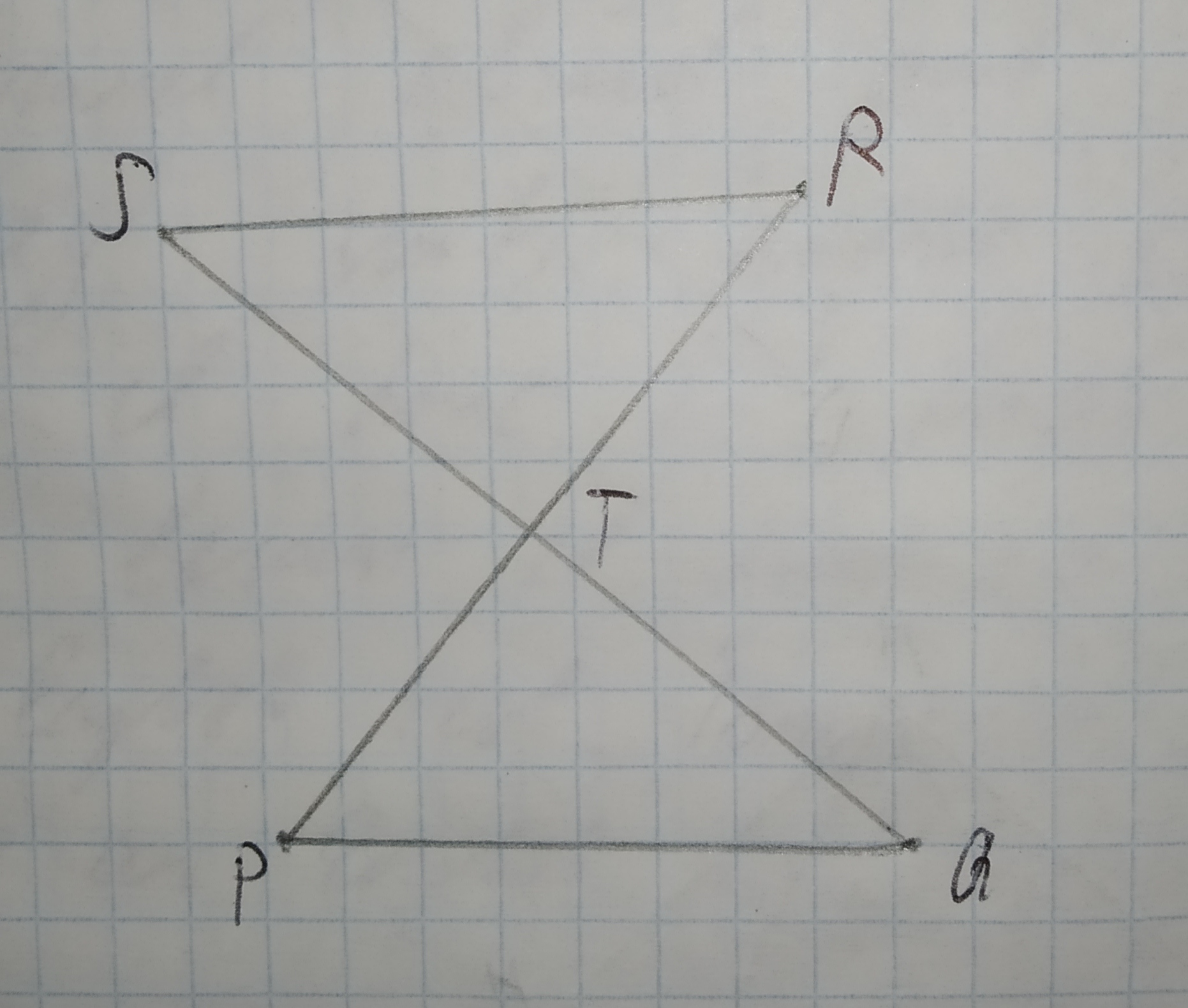Given: bar(SQ) and bar(PR) bisect each other.State the third congruence required to prove the congruence of triangles using the indicated postulate.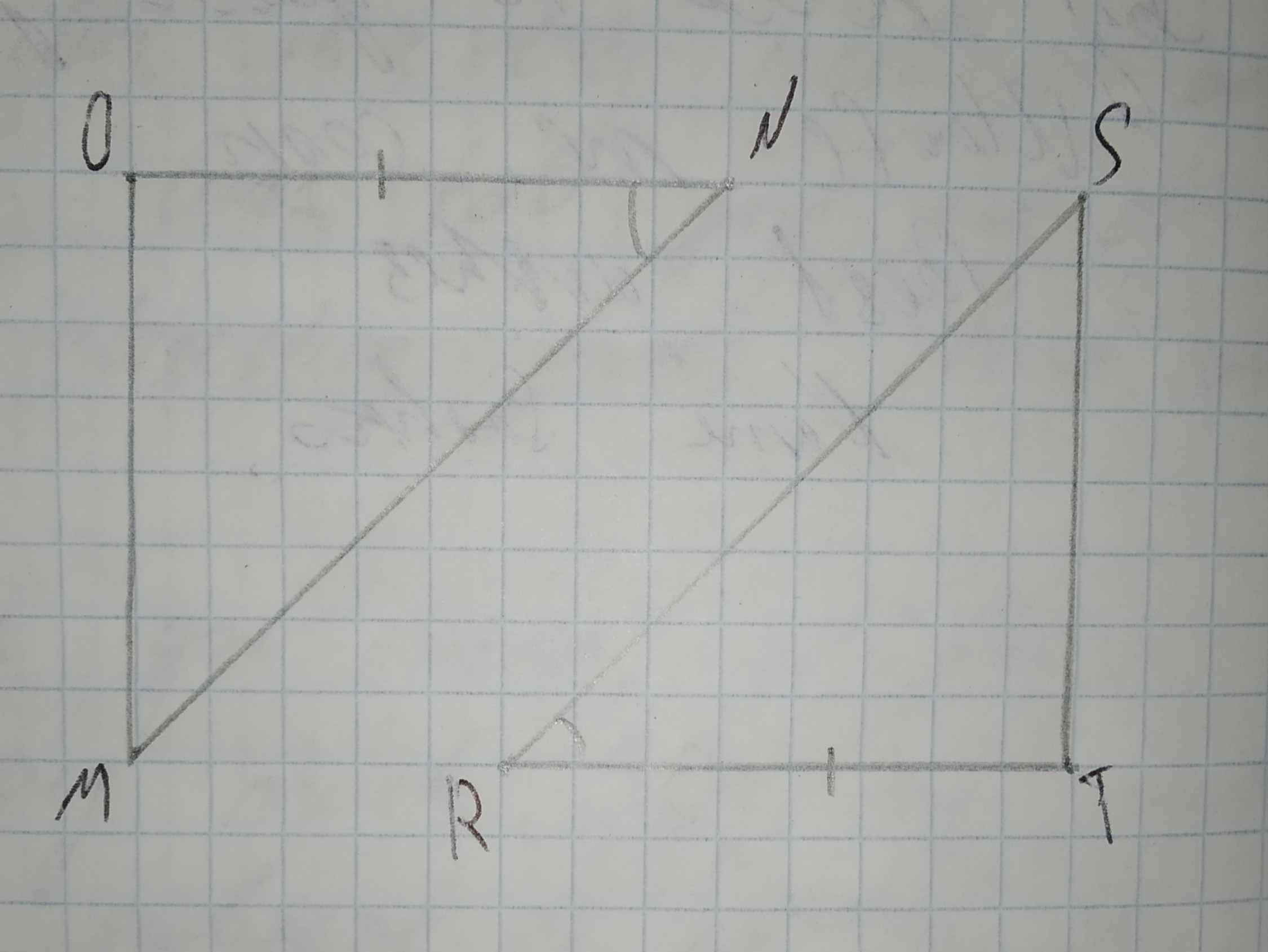a)$$\displaystyle\overline{{{O}{M}}}\stackrel{\sim}{=}\overline{{{T}{S}}}$$
b)$$\displaystyle\angle{M}\stackrel{\sim}{=}\angle{S}$$
c)$$\displaystyle\overline{{{O}{N}}}\stackrel{\sim}{=}\overline{{{T}{R}}}$$
d)$$\displaystyle\angle{O}\stackrel{\sim}{=}\angle{T}$$What other information do you need in order to prove the triangles congruent using the SAS Congruence Postulate?A)$$\displaystyle\angle{B}{A}{C}\stackrel{\sim}{=}\angle{D}{A}{C}$$
B)$$\displaystyle\overline{{{A}{C}}}\stackrel{\sim}{=}\overline{{{B}{D}}}$$
C)$$\displaystyle\angle{B}{C}{A}\stackrel{\sim}{=}\angle{D}{C}{A}$$
D)$$\displaystyle\overline{{{A}{C}}}\stackrel{\sim}{=}\overline{{{B}{D}}}$$State the third congruence required to prove the congruence of triangles using the indicated postulate.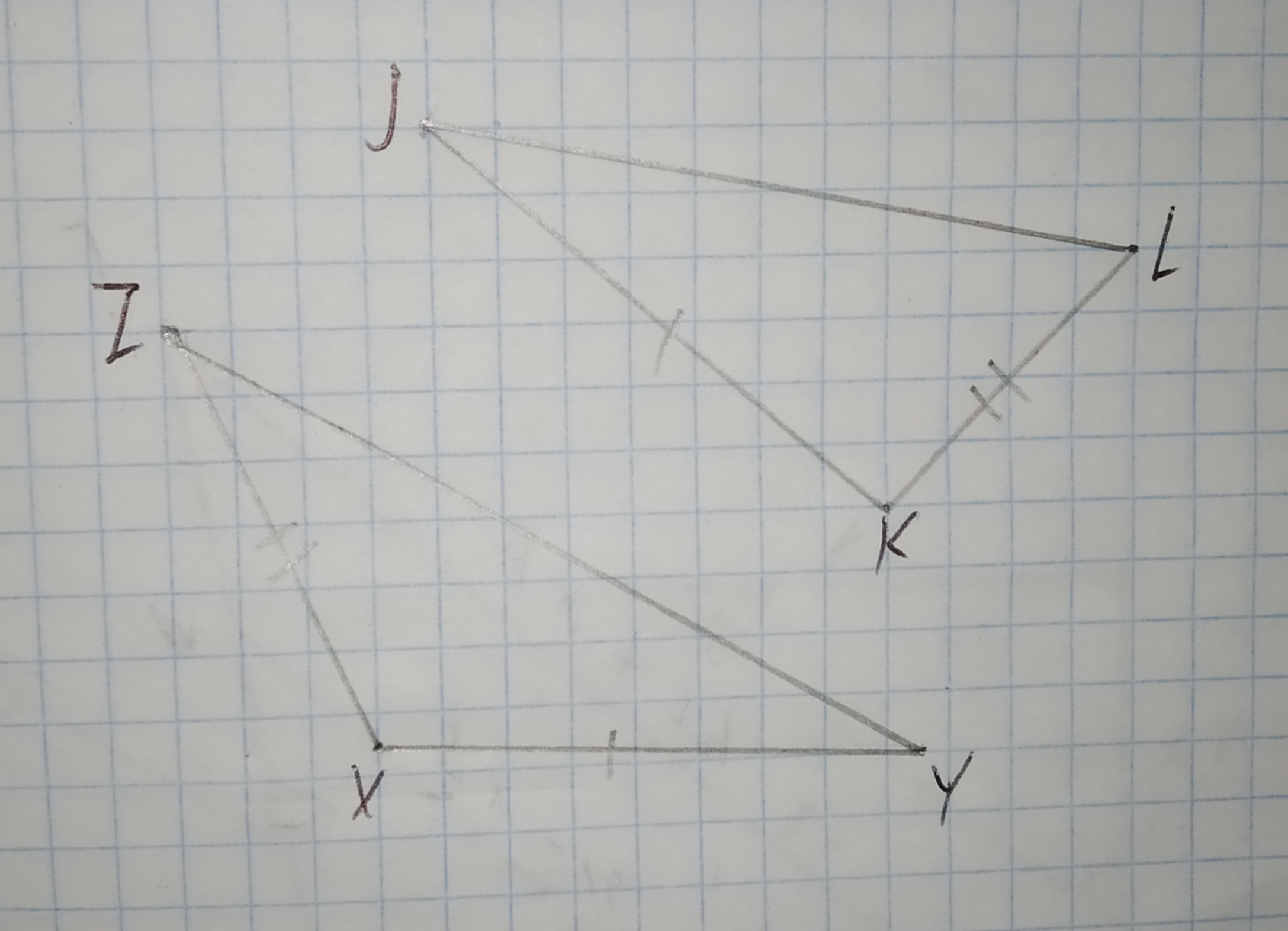a)$$\displaystyle\overline{{{Z}{Y}}}\stackrel{\sim}{=}\overline{{{J}{L}}}$$
b)$$\displaystyle\angle{X}\stackrel{\sim}{=}\angle{K}$$
c)$$\displaystyle\overline{{{K}{L}}}\stackrel{\sim}{=}\overline{{{X}{Z}}}$$
d)$$\displaystyle\angle{Y}\stackrel{\sim}{=}\angle{L}$$Select all statements that are true about the triangles.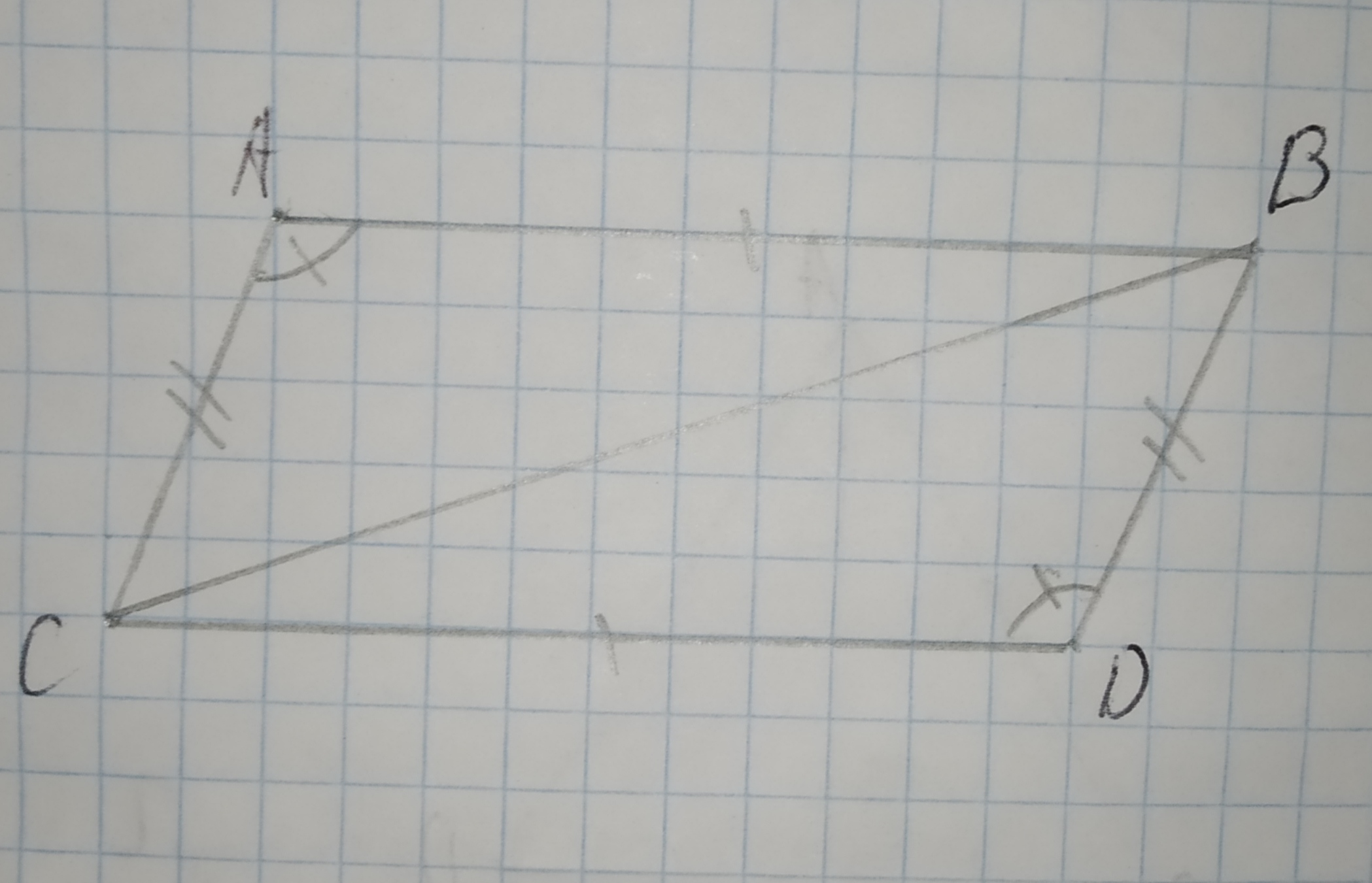-Triangle ABC and DCB are congruent by the Angle-Angle Triangle Congruence theorem.
-Triangle ABC and BCD are congruent by the Angle-Side-Angle Triangle Congruence theorem.
-Triangle ABC and BCD are congruent by the Side-Side-Side Triangle Congruence theorem.
-Triangle ABC and DCB are congruent by the Side-Angle-Side Triangle Congruence theorem.
-Triangle ABC and DCB are congruent by the Side-Side-Side Triangle Congruence theorem.
-There is not enough information to determine if the triangles are congruent.decide whether enough information is given to prove that the triangles are congruent using the SAS Congruence Theorem.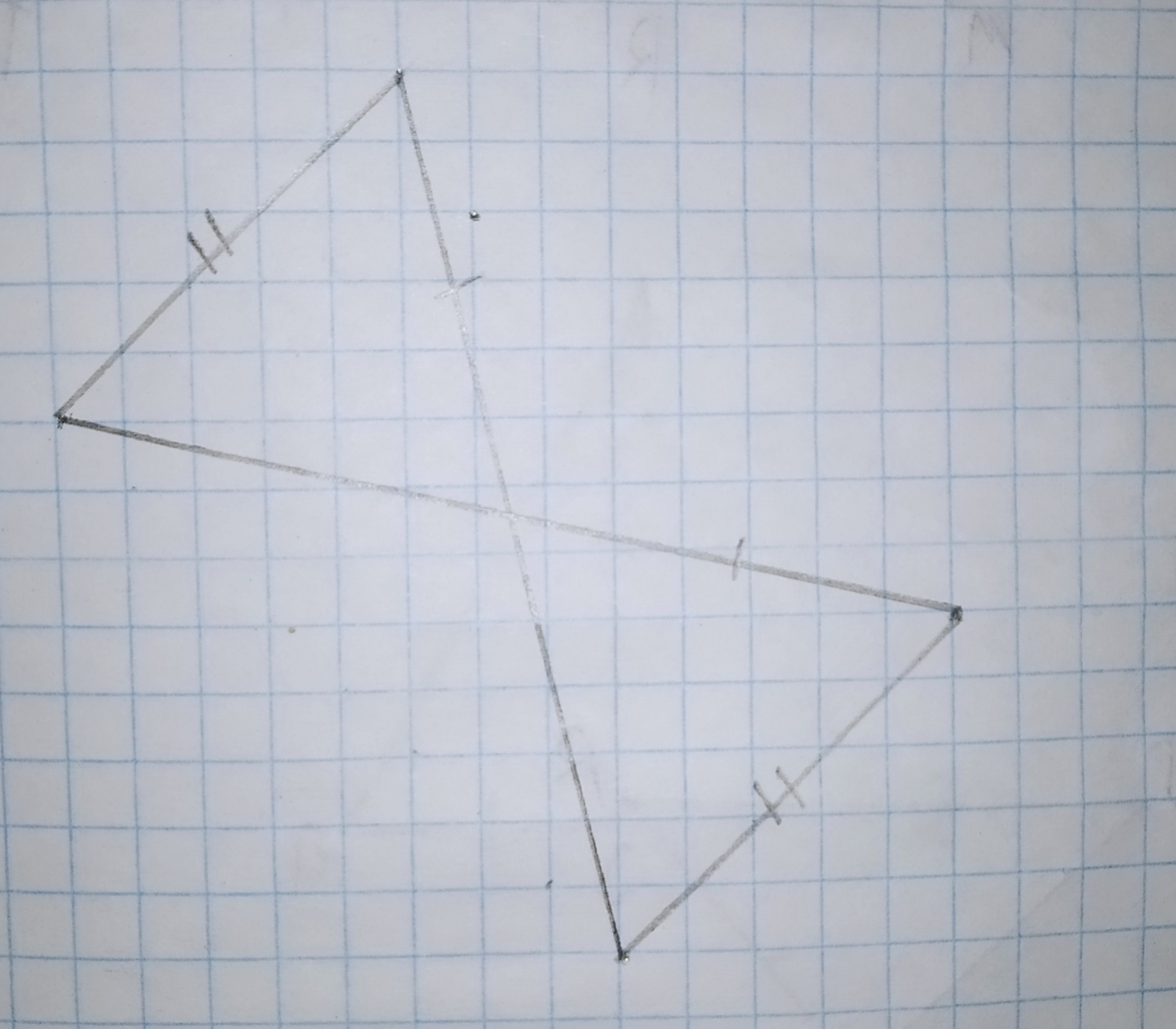Decide whether enough information is given to prove that the triangles are congruent using the SAS Congruence Theorem.